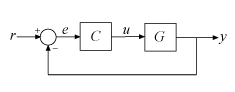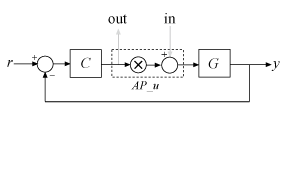Documentation

### This is machine translation

Mouseover text to see original. Click the button below to return to the English version of the page.

# connect

Block diagram interconnections of dynamic systems

## Syntax

```sysc = connect(sys1,...,sysN,inputs,outputs) sysc = connect(sys1,...,sysN,inputs,outputs,APs) sysc = connect(blksys,connections,inputs,outputs) sysc = connect(___,opts) ```

## Description

`sysc = connect(sys1,...,sysN,inputs,outputs)` connects the block diagram elements `sys1,...,sysN` based on signal names. The block diagram elements `sys1,...,sysN` are dynamic system models. These models can include summing junctions that you create using `sumblk`. The `connect` command interconnects the block diagram elements by matching the input and output signals that you specify in the `InputName` and `OutputName` properties of `sys1,...,sysN`. The aggregate model `sysc` is a dynamic system model having inputs and outputs specified by `inputs` and `outputs` respectively.

`sysc = connect(sys1,...,sysN,inputs,outputs,APs)` inserts an `AnalysisPoint` at every signal location specified in `APs`. Use analysis points to mark locations of interest which are internal signals in the aggregate model. For instance, a location at which you want to extract a loop transfer function or measure the stability margins is a location of interest.

`sysc = connect(blksys,connections,inputs,outputs)` uses index-based interconnection to build `sysc` out of an aggregate, unconnected model `blksys`. The matrix `connections` specifies how the outputs and inputs of `blksys` interconnect. For index-based interconnections, `inputs` and `outputs` are index vectors that specify which inputs and outputs of `blksys` are the external inputs and outputs of `sysc`. This syntax can be convenient when you do not want to assign names to all inputs and outputs of all models to connect. However, in general, it is easier to keep track of named signals.

`sysc = connect(___,opts)` builds the interconnected model using additional options. You can use `opts` with the input arguments of any of the previous syntaxes.

## Input Arguments

 `sys1,...,sysN` Dynamic system models that correspond to the elements of your block diagram. For example, the elements of your block diagram can include one or more `tf` or `ss` models that represent plant dynamics. Block diagram elements can also include a `pid` or `tunablePID` model representing a controller. You can also include one or more summing junction that you create using `sumblk`. Provide multiple arguments `sys1,...,sysN` to represent all of the block diagram elements and summing junctions. `inputs` For name-based interconnection, a character vector or cell array of character vectors that specify the inputs of the aggregate model `sysc`. The inputs in `inputs` must correspond to entries in the `InputName` or `OutputName` property of one or more of the block diagram elements `sys1,...,sysN`. `outputs` For name-based interconnection, a character vector or cell array of character vectors that specify the outputs of the aggregate model `sysc`. The outputs in `outputs` must correspond to entries in the `OutputName` property of one or more of the block diagram elements `sys1,...,sysN`. `APs` Locations (internal signals) of interest in the aggregate model, specified as a character vector or cell array of character vectors, such as `'X'` or `{'AP1','AP2'}`. The resulting model contains an analysis point at each such location. (See `AnalysisPoint`). Each location in `APs` must correspond to an entry in the `InputName` or `OutputName` property of one or more of the block diagram elements `sys1,...,sysN`. `blksys` Unconnected aggregate model. To obtain `blksys`, use `append` to join dynamic system models of the elements of your block diagram. For example, if your block diagram contains dynamic system models `C`, `G`, and `S`, create `blksys` with the following command: `blksys = append(C,G,S)` `connections` Matrix that specifies the connections and summing junctions of the block diagram. Each row of `connections` specifies one connection or summing junction in terms of the input vector `u` and output vector `y` of the unconnected aggregate model `blksys`. For example, the row: `[3 2 0 0]` specifies that `y(2)` connects into `u(3)`. The row ```[7 2 -15 6] ``` indicates that `y(2)-y(15)+y(6)` feeds into `u(7)`. If you do not specify any connection for a particular input or output, `connect` omits that input or output from the aggregate model. `opts` Additional options for interconnection, specified as an options set that you create with `connectOptions`.

## Output Arguments

 `sysc` Interconnected system, returned as either a state-space model or frequency-response model. The type of model returned depends on the input models. For example: Interconnecting numeric LTI models (other than `frd` models) returns an `ss` model.Interconnecting a numeric LTI model with a Control Design Block returns a generalized LTI model. For instance, interconnecting a `tf` model with a `tunablePID` Control Design Block returns a `genss`.Interconnecting any model with frequency-response data model returns a frequency response data model. By default, `connect` automatically discards states that do not contribute to the I/O transfer function from the specified inputs to the specified outputs of the interconnected model. To retain the unconnected states, set the `Simplify` option of `connectOptions` to `false`. For example: ```opt = connectOptions('Simplify',false); sysc = connect(sys1,sys2,sys3,'r','y',opt);```

## Examples

### SISO Feedback Loop

Create an aggregate model of the following block diagram from `r` to `y`.Create `C` and `G`, and name the inputs and outputs.

```C = pid(2,1); C.u = 'e'; C.y = 'u'; G = zpk([],[-1,-1],1); G.u = 'u'; G.y = 'y'; ```

The notations `C.u` and `C.y` are shorthand expressions equivalent to `C.InputName` and `C.OutputName`, respectively. For example, entering `C.u = 'e'` is equivalent to entering `C.InputName = 'e'`. The command sets the `InputName` property of `C` to the value `'e'`.

Create the summing junction.

```Sum = sumblk('e = r - y'); ```

Combine `C`, `G`, and the summing junction to create the aggregate model from `r` to `y`.

`T = connect(G,C,Sum,'r','y');`

`connect` automatically joins inputs and outputs with matching names.

### MIMO Feedback Loop

Create the control system of the previous example where `G` and `C` are both 2-input, 2-output models.

```C = [pid(2,1),0;0,pid(5,6)]; C.InputName = 'e'; C.OutputName = 'u'; G = ss(-1,[1,2],[1;-1],0); G.InputName = 'u'; G.OutputName = 'y';```

When you specify single names for vector-valued signals, the software automatically performs vector expansion of the signal names. For example, examine the names of the inputs to `C`.

`C.InputName`
```ans = 'e(1)' 'e(2)'```

Create a 2-input, 2-output summing junction.

`Sum = sumblk('e = r-y',2);`

`sumblk` also performs vector expansion of the signal names.

Interconnect the models to obtain the closed-loop system.

`T = connect(G,C,Sum,'r','y');`

The block diagram elements `G`, `C`, and `Sum` are all 2-input, 2-output models. Therefore, `connect` performs the same vector expansion. `connect` selects all entries of the two-input signals `'r'` and `'y'` as inputs and outputs to `T`, respectively. For example, examine the input names of `T`.

`T.InputName`
```ans = 'r(1)' 'r(2)'```

### Feedback Loop With Analysis Point Inserted by `connect`

Create a model of the following block diagram from r to y. Insert an analysis point at an internal location, u.Create `C` and `G`, and name the inputs and outputs.

```C = pid(2,1); C.InputName = 'e'; C.OutputName = 'u'; G = zpk([],[-1,-1],1); G.InputName = 'u'; G.OutputName = 'y';```

Create the summing junction.

`Sum = sumblk('e = r - y');`

Combine `C`, `G`, and the summing junction to create the aggregate model, with an analysis point at u.

`T = connect(G,C,Sum,'r','y','u')`
```T = Generalized continuous-time state-space model with 1 outputs, 1 inputs, 3 states, and the following blocks: AnalysisPoints_: Analysis point, 1 channels, 1 occurrences. Type "ss(T)" to see the current value, "get(T)" to see all properties, and "T.Blocks" to interact with the blocks. ```

The resulting `T` is a `genss` model. The `connect` command creates the `AnalysisPoint` block, `AnalysisPoints_`, and inserts it into `T`. To see the name of the analysis point channel in `AnalysisPoints_`, use `getPoints`.

`getPoints(T)`
```ans = 1x1 cell array {'u'} ```

The analysis point channel is named `'u'`. You can use this analysis point to extract system responses. For example, the following commands extract the open-loop transfer at u and the closed-loop response at y to a disturbance injected at u.

```L = getLoopTransfer(T,'u',-1); Tuy = getIOTransfer(T,'u','y');```

`T` is equivalent to the following block diagram, where AP_u designates the `AnalysisPoint` block `AnalysisPoints_` with channel name u.### Index-Based Interconnection

Create an aggregate model of the following block diagram from `r` to `y` using index-based interconnection.Create `C`, `G`, and the unconnected aggregate model `blksys`.

```C = pid(2,1); G = zpk([],[-1,-1],1); blksys = append(C,G);```

The inputs `u(1),u(2)` of `blksys` correspond to the inputs of `C` and `G`, respectively. The outputs `w(1),w(2)` of `blksys` correspond to the outputs of `C` and `G`, respectively.

Create the matrix `connections`, which specifies which outputs of `blksys` connect to which inputs of `blksys`.

`connections = [2 1; 1 -2];`

The first row indicates that `w(1)` connects to `u(2)`; in other words, that the output of `C` connects to the input of `G`. The second row indicates that `-w(2)` connects to `u(1)`; in other words, that the negative of the output of `G` connects to the input of `C`.

Create the connected aggregate model from `r` to `y`.

```T = connect(blksys,connections,1,2) ```

The last two arguments specify the external inputs and outputs in terms of the indices of `blksys`. The argument `1` specifies that the external input connects to `u(1)`. The last argument, `2`, specifies that the external output connects from `w(2)`.